Скачать презентацию Series Method of Differences also known as telescoping

04 Method of Differences.pptx

• Количество слайдов: 12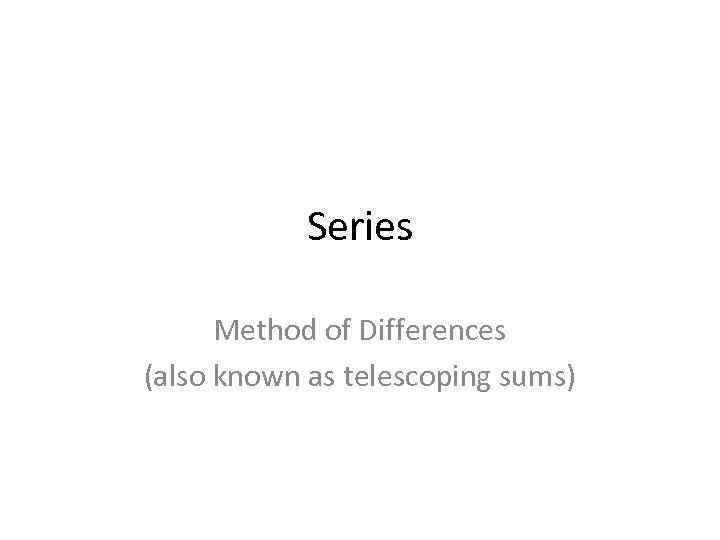Series Method of Differences (also known as telescoping sums)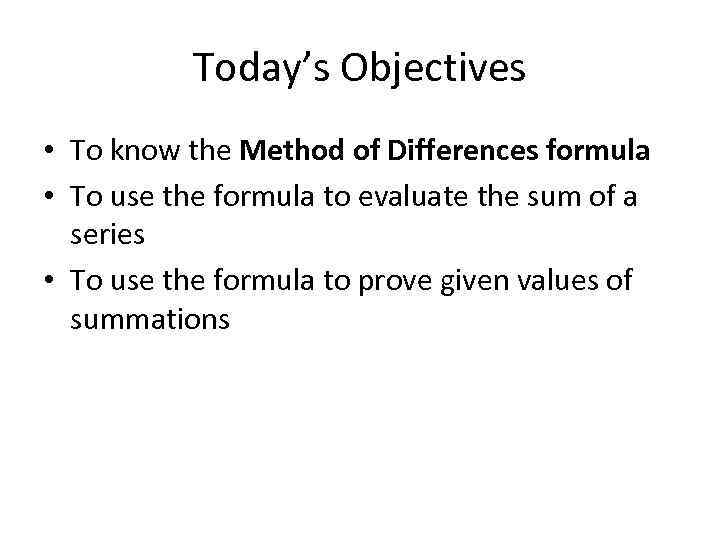Today’s Objectives • To know the Method of Differences formula • To use the formula to evaluate the sum of a series • To use the formula to prove given values of summations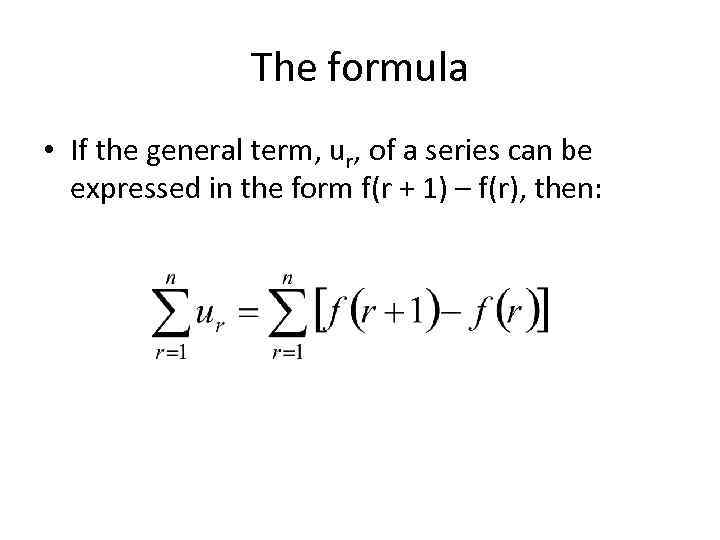The formula • If the general term, ur, of a series can be expressed in the form f(r + 1) – f(r), then: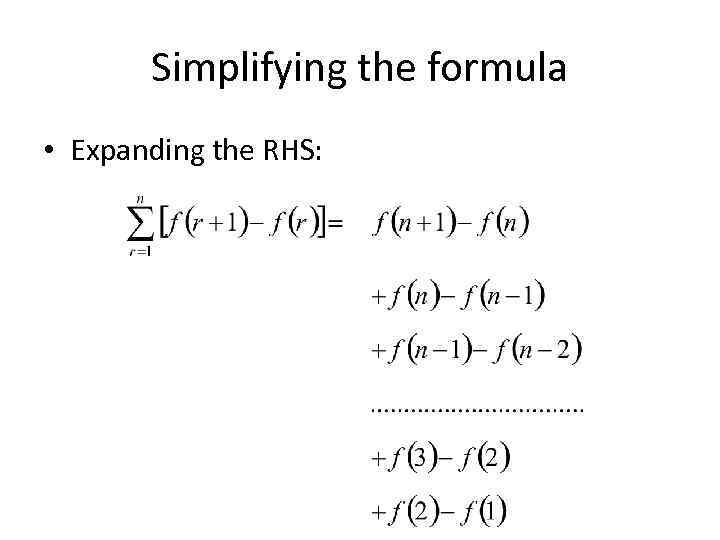Simplifying the formula • Expanding the RHS: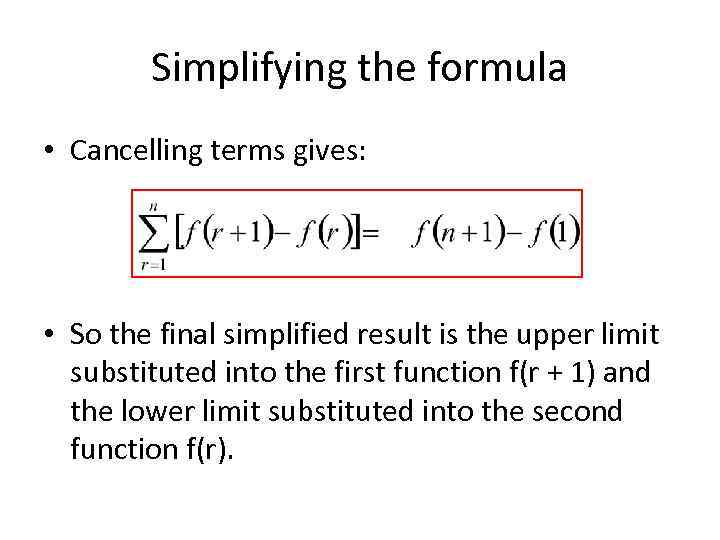Simplifying the formula • Cancelling terms gives: • So the final simplified result is the upper limit substituted into the first function f(r + 1) and the lower limit substituted into the second function f(r).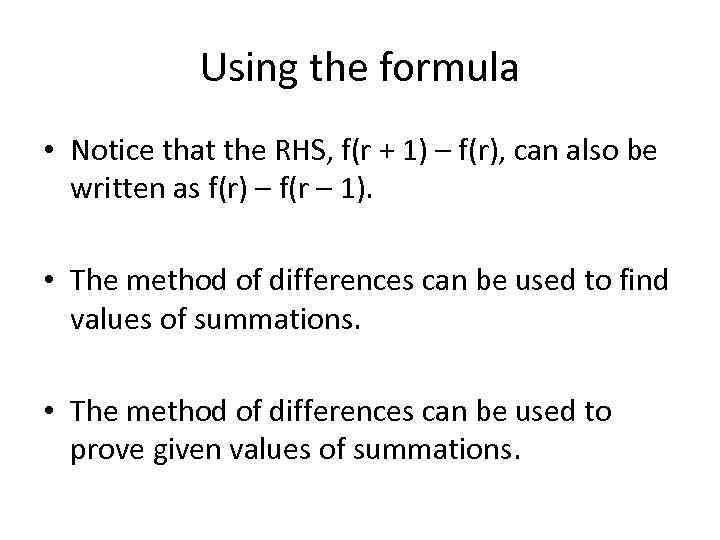Using the formula • Notice that the RHS, f(r + 1) – f(r), can also be written as f(r) – f(r – 1). • The method of differences can be used to find values of summations. • The method of differences can be used to prove given values of summations.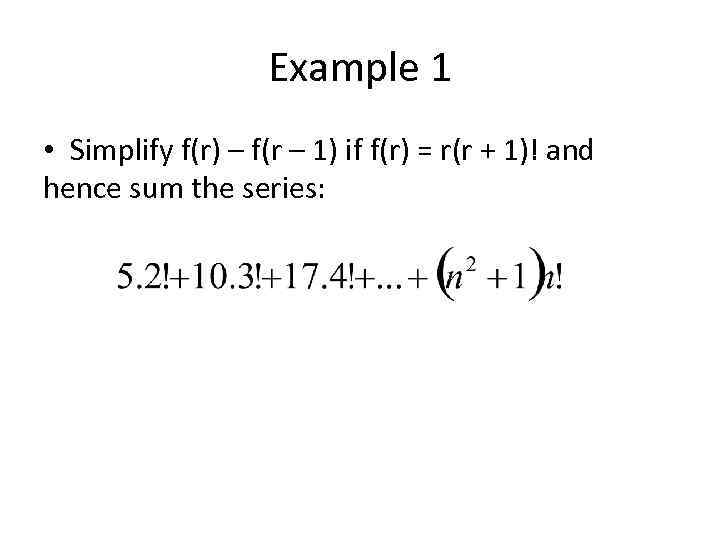Example 1 • Simplify f(r) – f(r – 1) if f(r) = r(r + 1)! and hence sum the series: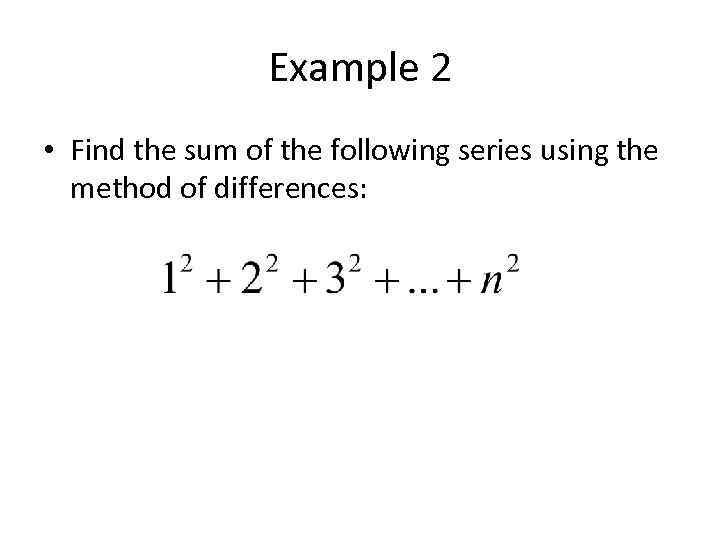Example 2 • Find the sum of the following series using the method of differences: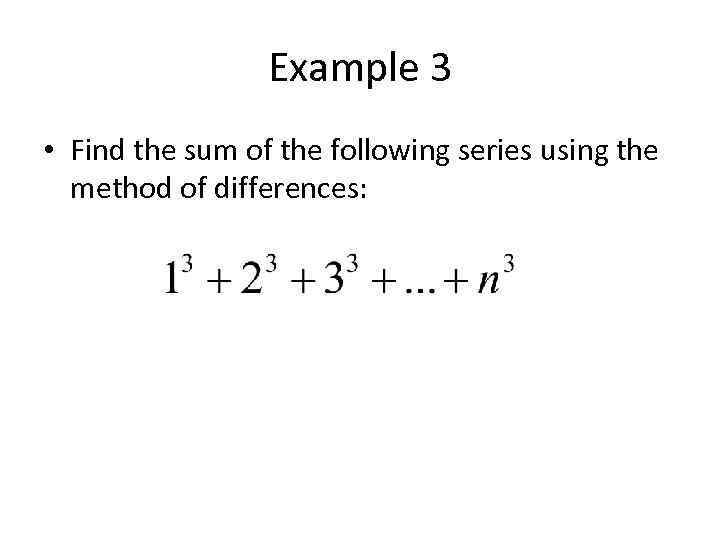Example 3 • Find the sum of the following series using the method of differences: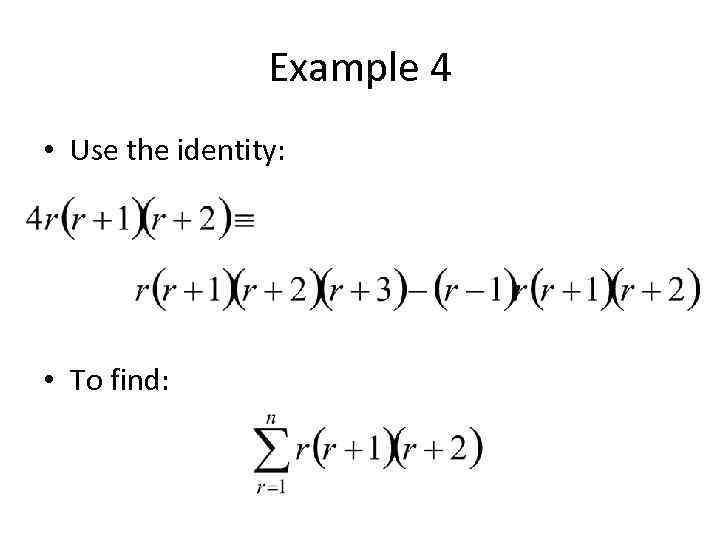Example 4 • Use the identity: • To find: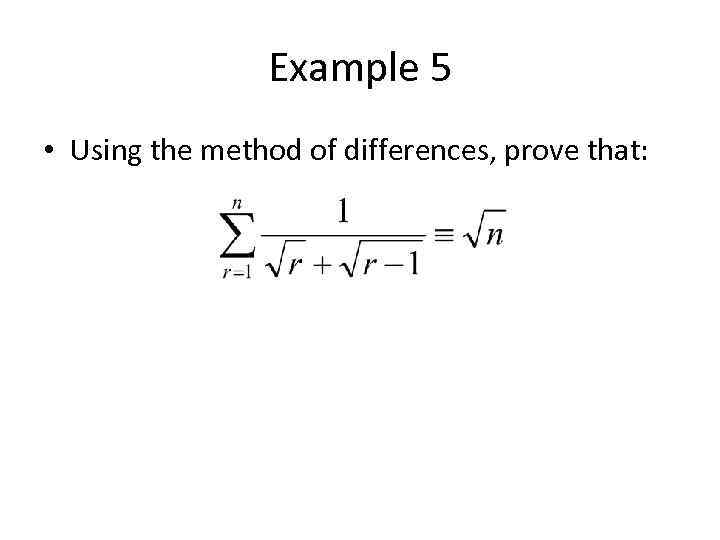Example 5 • Using the method of differences, prove that: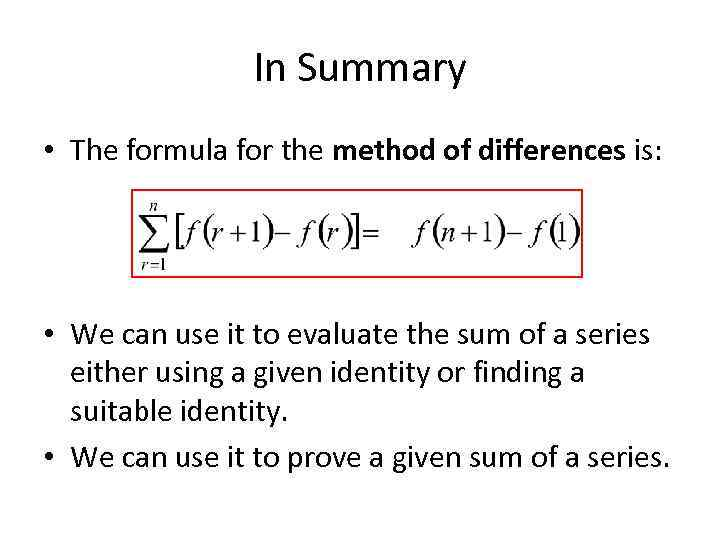In Summary • The formula for the method of differences is: • We can use it to evaluate the sum of a series either using a given identity or finding a suitable identity. • We can use it to prove a given sum of a series.